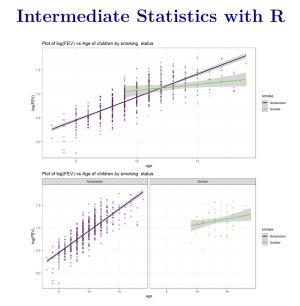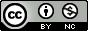# Intermediate Statistics with R

by Mark C. GreenwoodJoin

### Book Description

Introductory statistics courses prepare students to think statistically but cover relatively few statistical methods. Building on the basic statistical thinking emphasized in an introductory course, a second course in statistics at the undergraduate level can explore a large number of statistical methods. This text covers more advanced graphical summaries, One-Way ANOVA with pair-wise comparisons, Two-Way ANOVA, Chi-square testing, and simple and multiple linear regression models. Models with interactions are discussed in the Two-Way ANOVA and multiple linear regression setting with categorical explanatory variables. Randomization-based inferences are used to introduce new parametric distributions and to enhance understanding of what evidence against the null hypothesis "looks like". Throughout, the use of the statistical software R via Rstudio is emphasized with all useful code and data sets provided within the text.

Chapter 1
Preface
Chapter 2
(R)e-Introduction to statistics
Chapter 3
One-Way ANOVA
Chapter 4
Two-Way ANOVA
Chapter 5
Chi-square tests
Chapter 6
Correlation and Simple Linear Regression
Chapter 7
Simple linear regression inference
Chapter 8
Multiple linear regression
Chapter 9
Case studies

### Book Details

Title
Intermediate Statistics with R
Subject
Computer Science
Publisher
Montana State University
Published
2021
Pages
428
Edition
1
Language
English
PDF Size
7.8 MB### Related BooksThis ICME-13 Topical Survey provides a review of recent research into statistics education, with a focus on empirical research published in established educational journals and on the proceedings of important conferences on statistics education. It identifies and addresses six key research topics, namely: teachers' knowledge; teachers' ro...If you know how to program with Python and also know a little about probability, you're ready to tackle Bayesian statistics. With this book, you'll learn how to solve statistical problems with Python code instead of mathematical notation, and use discrete probability distributions instead of continuous mathematics. Once you get the math o...Ccurrently many of Julia's users are hard-core developers that contribute to the language's standard libraries, and to the extensive package eco-system that surrounds it. Therefore, much of the Julia material available at present is aimed at other developers rather than end users. This is where our book comes in, as it has been written wi...The demand for skilled data science practitioners in industry, academia, and government is rapidly growing. This book introduces concepts and skills that can help you tackle real-world data analysis challenges. It covers concepts from probability, statistical inference, linear regression and machine learning. It also helps you develop skills such a...This open access textbook provides the background needed to correctly use, interpret and understand statistics and statistical data in diverse settings. Part I makes key concepts in statistics readily clear. Parts I and II give an overview of the most common tests (t-test, ANOVA, correlations) and work out their statistical principles. Part III pr...The revised 2nd edition of this book provides the reader with a solid foundation in probability theory and statistics as applied to the physical sciences, engineering and related fields. It covers a broad range of numerical and analytical methods that are essential for the correct analysis of scientific data, including probability theory, distribut...